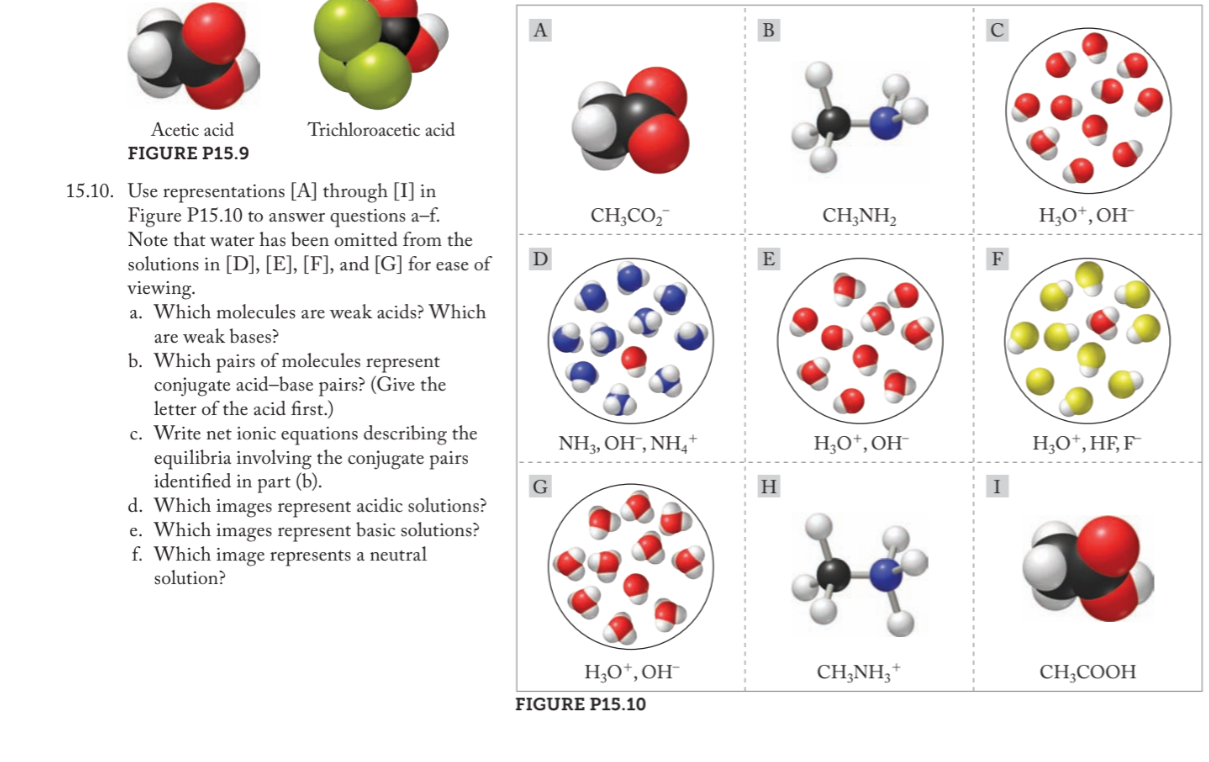# Use representations [A] through [I] in Figure P15.10 to answer questions a-f. Note that water has been omitted from the solutions in [D], [E], [F], and [G] for ease of viewing. a. Which molecules are weak acids? Which are weak bases b. Which pairs of molecules represent conjugate acid-base pairs? (Give the letter of the acid first.) c. Write net ionic equations describing equilibria involving the conjugate pairs identified in part (b) d. Which images represent acidic solutions? e. Which images represent basic solutions? f. Which image represents a neutral solution?